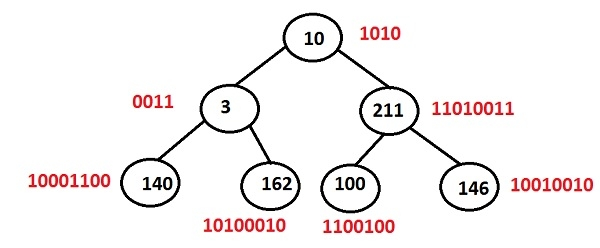# Print the number of set bits in each node of a Binary Tree in C++ Programming.

Given the binary tree, the function will generate the binary values of the keys stored in the nodes and then return the number of set bits(1) in that binary equivalent.## Example

Binary tree having keys as: 10 3 211 140 162 100 and 146

KeyBinary equivalentSet bits(output)
1010102
300112
211110100115
140100011003
162101000103
10011001003
146100100103

Here we are using the function __builtin_popcount

The function prototype is as follows −

int __builtin_popcount(unsigned int)

It returns the numbers of set bits in an integer i.e. the number of ones in the binary representation of the integer.

## Algorithm

START
Step 1 -> create a structure of a node as
struct Node
struct node *left, *right
int data
End
Step 2 -> function to create a node
node* newnode(int data)
node->data = data
node->left = node->right = NULL;
return (node)
Step 3 -> Create function for generating bits of a node data
void bits(Node* root)
IF root = NULL
return
print __builtin_popcount(root->data)
bits(root->left)
bits(root->right)
step 4 -> In main()
create tree using Node* root = newnode(10)
root->left = newnode(3)
call bits(root)
STOP

## Example

Live Demo

#include <bits/stdc++.h>
using namespace std;
// structure of a node
struct Node {
int data;
struct Node *left, *right;
};
//function to create a new node
Node* newnode(int data) {
Node* node = new Node;
node->data = data;
node->left = node->right = NULL;
return (node);
}
//function for finding out the node
void bits(Node* root){
if (root == NULL)
return;
//__builtin_popcount counts the number of set bit of a current node
cout << "bits in node " << root->data << " = " <<__builtin_popcount(root->data)<< "\n";
bits(root->left);
bits(root->right);
}
int main(){
Node* root = newnode(10);
root->left = newnode(3);
root->left->left = newnode(140);
root->left->right = newnode(162);
root->right = newnode(211);
root->right->left = newnode(100);
root->right->right = newnode(146);
bits(root);
return 0;
}

## Output

if we run the above program then it will generate the following output

bits in node 10 = 2
bits in node 3 = 2
bits in node 140 = 3
bits in node 162 = 3
bits in node 211 = 5
bits in node 100 = 3
bits in node 146 = 3